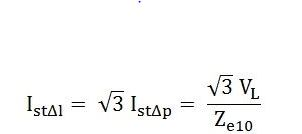# Star Delta Starter? Working principle,Theory, Circuit Diagram

0
471

## Working Principle Of Star – Delta Starter:

### Connection diagram of star – delta starter:#### During start position of the switch, the stator windings are connected in the star as shown in the following figure.#### Now the induction motor gradually picks up its speed. When the speed becomes 80 percent of its rated speed then the switch moves to run position as a result stator windings get connected in delta as shown in the below figure.## Theory And Calculations Of Star – Delta Starter:

#### V1 is the phase voltage.

Istyp be starting current per phase when the stator windings are connected in star.

#### Istyl is the starting line current when the stator windings are connected in star.

IstΔp is the starting current per phase by direct switching with the stator windings connected in delta
IstΔl is the starting line current by direct switching with the stator windings in the delta.
IscΔp is the short circuit phase current by direct switching with the stator windings in the delta.
Ze10 is the standstill equivalent impedance per phase of the motor, referred to the stator

#### In star connection,  line current will be equal to phase current.#### In the delta connection, the line current is equal to the √3 times of the phase current. so we get,#### This shows that with star delta starter, the starting current from the main supply is one-third of that with direct switching in the delta.#### This shows with star delta starter, the starting torque is reduced to one-third of the starting torque obtained with the direct switching in the delta.#### Here,